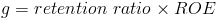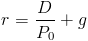Custom Search

# Present Value of Stock - Constant Growth

PV of Stock with Constant Growth Calculator (Click Here or Scroll Down)The formula for the present value of a stock with constant growth is the estimated dividends to be paid divided by the difference between the required rate of return and the growth rate.

The present value of a stock with constant growth is one of the formulas used in the dividend discount model, specifically relating to stocks that the theory assumes will grow perpetually. The dividend discount model is one method used for valuing stocks based on the present value of future cash flows, or earnings.

### How is the Present Value of Stock with Constant Growth Derived?

As previously stated, the present value of a stock with constant growth is based on the dividend discount model, which sums the discount of each cash flow to its present value. The formula shown at the top of the page for stocks with constant growth uses the present value of a growing perpetuity formula, based on the underlying theoretical assumption that a stock will continue indefinitely, or in perpetuity. This assumption is not without scrutiny, however the present value of a growing perpetuity can be used as a comparable measure along with other stock valuation methods for companies that are stable and tend to have a calculable outcome of steady growth.

#### Growth Rate in the Present Value of Stock Formula

The growth rate used for calculating the present value of a stock with constant growth can be estimated asMultiplying the retention ratio by the return on equity can then be reduced to retained earnings divided average stockholder's equity.

It is important to note that in practice, growth can not be infinitely negative nor can it exceed the required rate of return. A fair amount of stock valuation requires non-mathematical inference to determine the appropriate method used.

##### Required Rate of Return in the Present Value of Stock Formula

The required rate of return variable in the formula for valuing a stock with constant growth can be determined by a few different methods.

One method for finding the required rate of return is to use the capital asset pricing model.The capital asset pricing model method looks at the risk of a stock relative to the risk of the market to determine the required rate of return based on the return on the market.

Another method that can be used is to determine the required rate of return based on the present value of dividends. This method also uses the present value of a growing perpetuity formula and rearranges the formula to calculate the required rate of return. After rearranging the formula, it is shown aswhich is the dividend yield + growth rate.

The arbitrage pricing theory can also be used which is similar to the capital asset pricing model but uses various risk factors and the betas for each risk factor to determine the total risk premium for the stock.

New to Finance?